# 2. 成果¶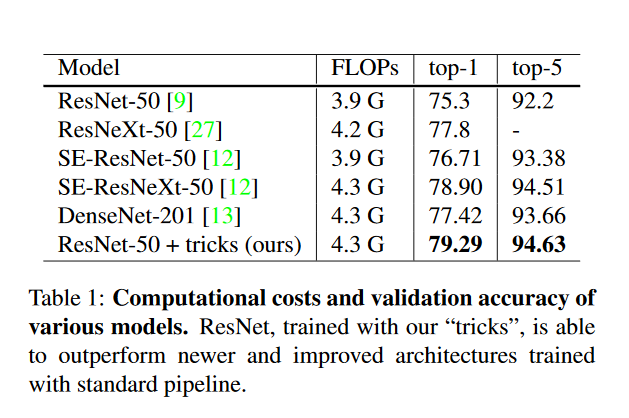# 2. BaseLine¶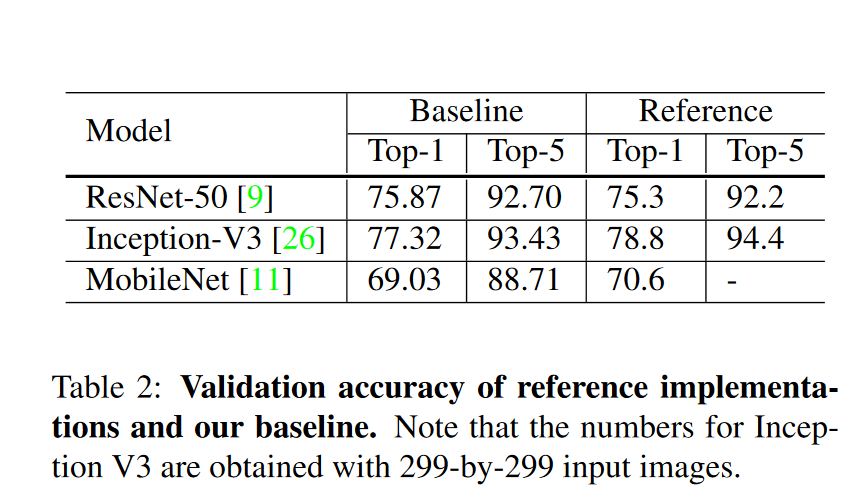# 3. 训练调参经验¶

## 3.1 模型训练加速¶

Accurate, Large Minibatch SGD: Training ImageNet in 1 Hour

• 增大学习率。因为更大的Batch Size意味着每个Batch数据计算得到的梯度更加贴近整个数据集，从数学上来说就是方差更好，因此当更新方向更加准确之后，迈的步子也可以更大，一般来说Batch Size变成原始几倍，学习率就增加几倍。
• Warm up。Warm up指的是用一个小的学习率先训练几个epoch，这是因为网络的参数是随机初始化的，假如一开始就采用较大的学习率容易出现数值不稳定，这也是为什么要使用Warm up。然后等到训练过程基本上稳定了就可以使用原始的初始学习率进行训练了。作者在使用Warm up的过程中使用线性增加的策略。举个例子假如Warm up阶段的初始学习率是0，warmup阶段共需要训练m个batch的数据（论文实现中m个batch共5个epoch），假设训练阶段的初始学习率是L，那么在第$i$个batch的学习率就设置为$i\times L / m$
• 每一个残差块后的最后一个BN层的$\gamma$参数初始化为0。我们知道BN层的$\gamma$$\beta$参数是用来对标注化后的数据做线性变换的，公式表示为：$y=\gamma x^+\beta$，其中我们一般会把$\gamma$设为1，而这篇论文提出初始化为$0$则更容易训练。
• 不对Bias参数做权重惩罚。对权重还是要做的。。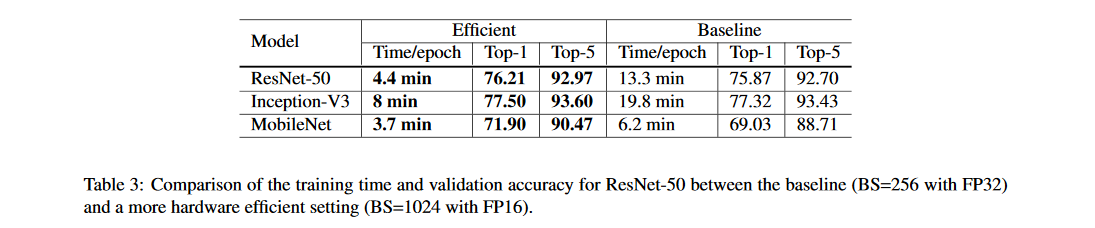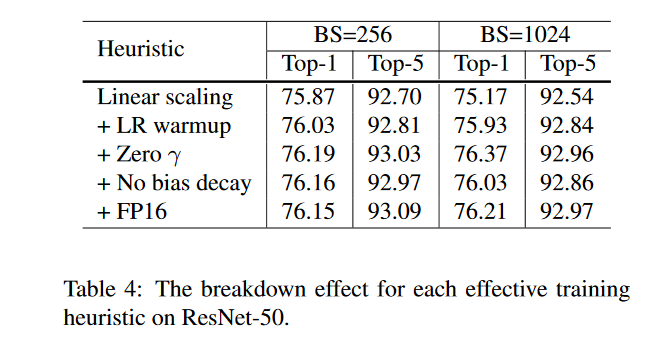## 3.2 网络结构调优¶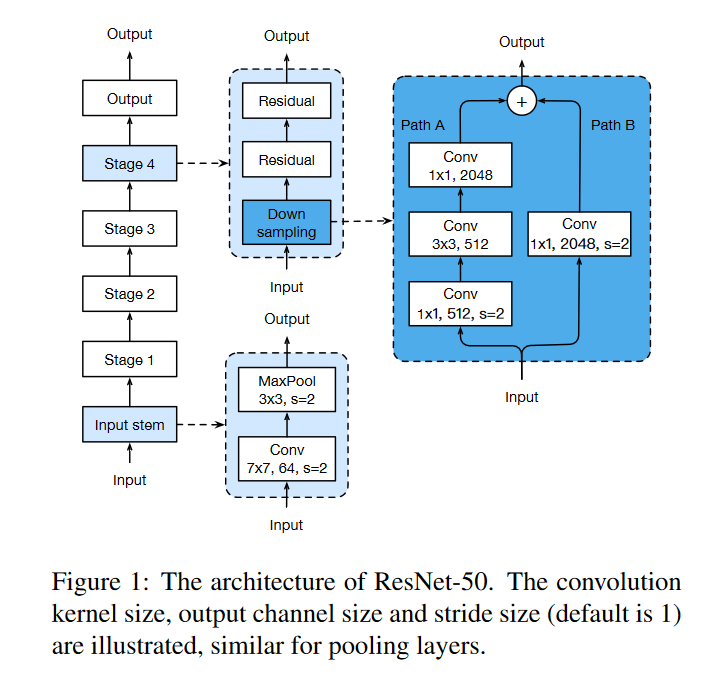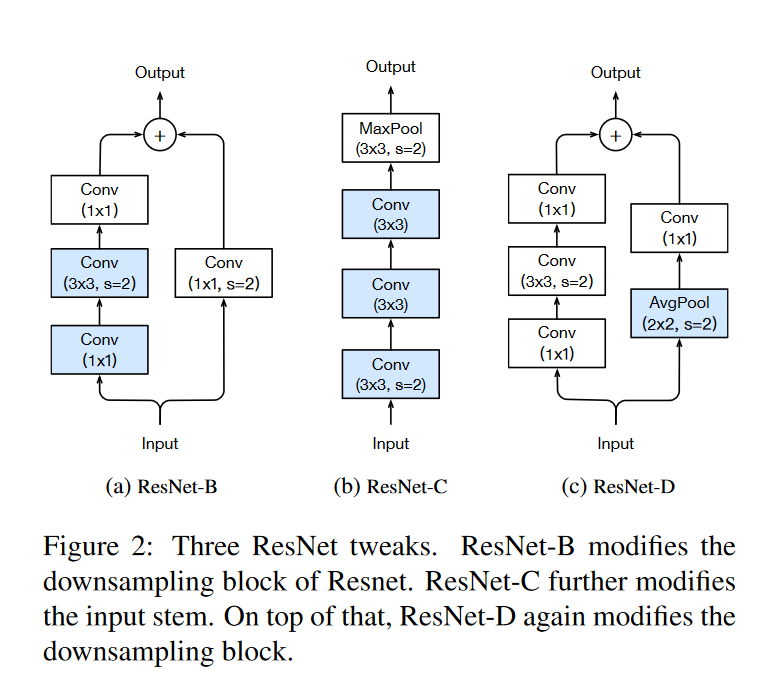• ResNet-B。ResNet-B改进的地方就是将4个Stage中做下采样的残差模块的下采样操作从第一个卷积层换到第三个卷积层，如果下采样操作放在$stride=2$$1\times 1$卷积层，那么会丢失比较多的特征信息(通道缩减默认是$\frac{1}{4}$)，而将下采样操作放在第$3$个卷积层则可以减少这种损失，因为即便$stride=2$，但是卷积核尺寸更大，保留有效信息更多。
• ResNet-C。ResNet-C改进的地方是将Figure1中的输入流部分的$7\times 7$卷积用两个$3\times 3$卷积来替换，这部分借鉴了Inception V2的思想，主要是为了减少运算量。但很遗憾，从后面的Table5可以看出$3$$3\times 3$卷积的FLOPS比原始的$7\times 7$+$3\times 3$卷积的FLOPS还多。。
• ResNet-D。ResNet-D改进的地方是将Stage部分做下采样的残差模块的支路从$stride=2$$1\times 1$卷积层换成$stride=1$的卷积层，并在前面添加一个池化层来做下采样。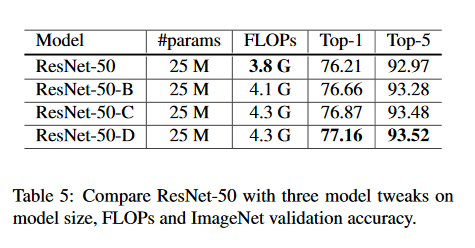## 3.3 模型训练调参¶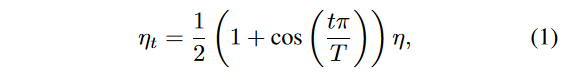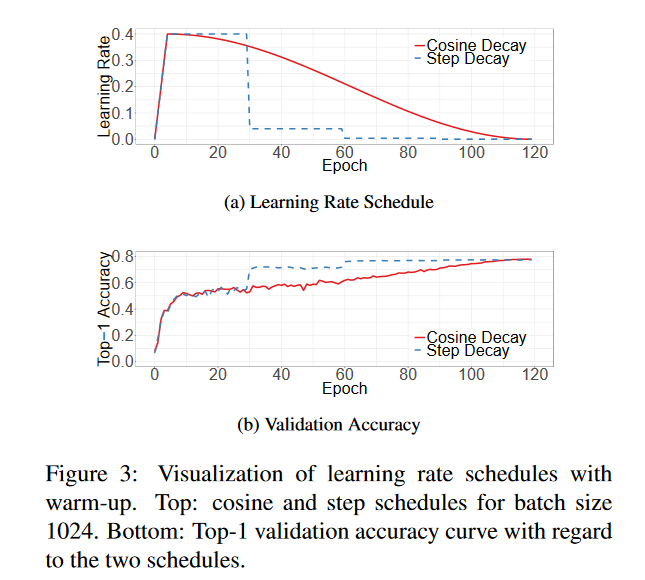• 使用标签平滑(label smooth)。这部分是把原始的one-hot类型标签做软化，这样可以在计算损失时一定程度的减小过拟合。从交叉熵损失函数可以看出，只有真实标签对应的类别概率才会对损失值计算有所帮助，因此标签平滑相当于减少真实标签的类别概率在计算损失值时的权重，同时增加其他类别的预测概率在最终损失函数中的权重。这样真实类别概率和其他类别的概率均值之间的gap（倍数）就会下降一些，Lable Smooth实际上就是下面的公式(4)。new_labels = (1.0 - label_smoothing) * one_hot_labels + label_smoothing / num_classes


import torch
import torch.nn as nn

class LabelSmoothing(nn.Module):
"""
NLL loss with label smoothing.
"""
def __init__(self, smoothing=0.0):
"""
Constructor for the LabelSmoothing module.
:param smoothing: label smoothing factor
"""
super(LabelSmoothing, self).__init__()
self.confidence = 1.0 - smoothing
self.smoothing = smoothing

def forward(self, x, target):
logprobs = torch.nn.functional.log_softmax(x, dim=-1)

nll_loss = -logprobs.gather(dim=-1, index=target.unsqueeze(1))
nll_loss = nll_loss.squeeze(1)
smooth_loss = -logprobs.mean(dim=-1)
loss = self.confidence * nll_loss + self.smoothing * smooth_loss
return loss.mean()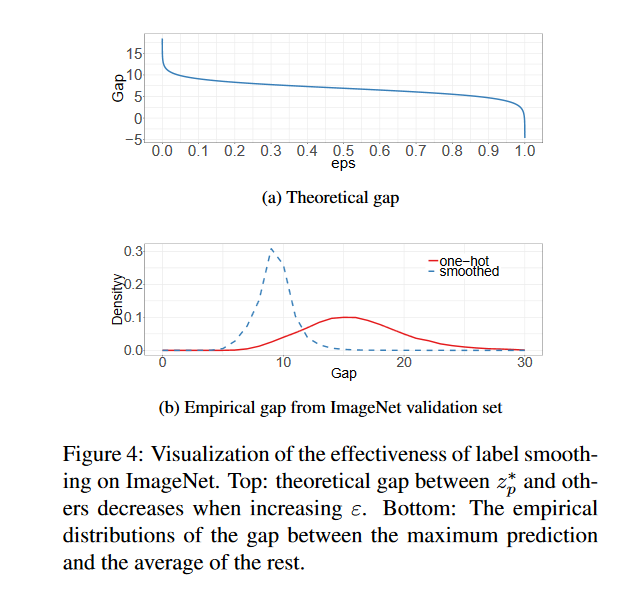• 知识蒸馏(knowledge distillation)。知识蒸馏时模型压缩领域的一个重要分支，即采用一个效果更好的teacher model训练student model，使得student model在模型结构不改变的情况下提升效果。这篇论文使用ResNet-152作为teacher model，用ResNet-50作student model。代码实现细节上，通过在ResNet网络后添加一个蒸馏损失函数实现，这个损失函数用来评价teacher model输出和student model输出的差异，因此整体的损失函数原损失函数和蒸馏损失函数的结合，如公式(6)所示：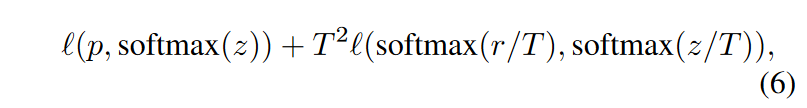• Mixup。论文还引入了Mixup这种数据增强方式，如果使用了Mixup数据增强来进行训练，那么每次需要读取2张输入图像，这里用$(x_i,y_i)$,$x_j,y_j$来表示，那么通过下面的公式就可以合成获得一张新的图像和标签$(\hat{x},\hat{y})$，然后使用张新图像进行训练，需要注意的是采用这种方式训练模型时要训更多epoch。式子中的$\lambda$是一个超参数，用来调节合成的比重，取值范围是$[0,1]$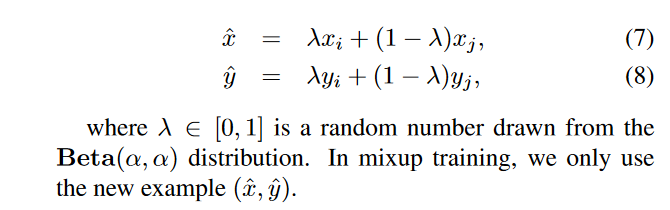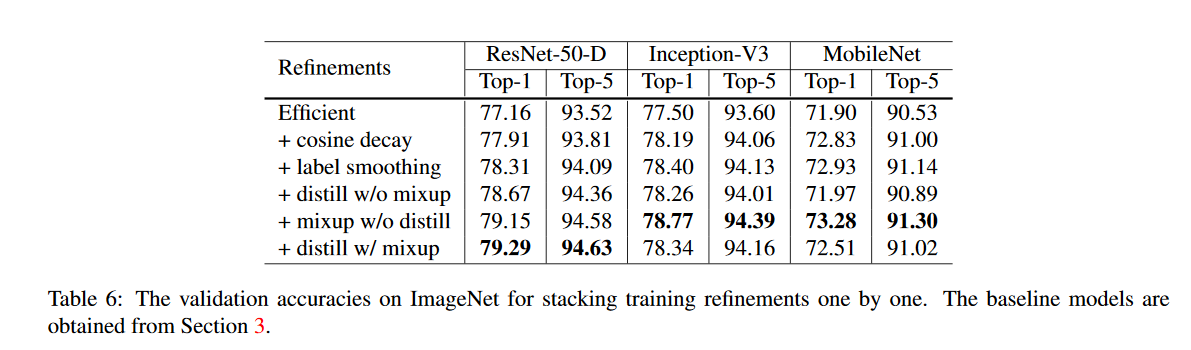# 4. 迁移学习¶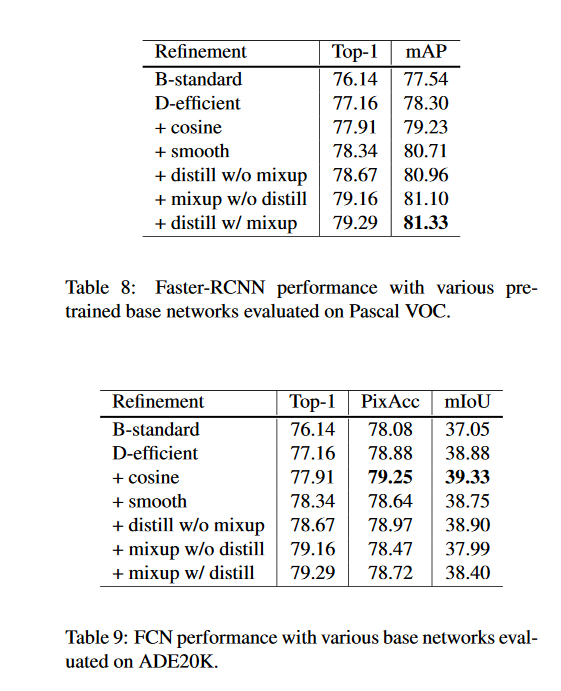# 7. 推荐阅读¶